# CO SWBAT generate the graphs of the sine

• Slides: 31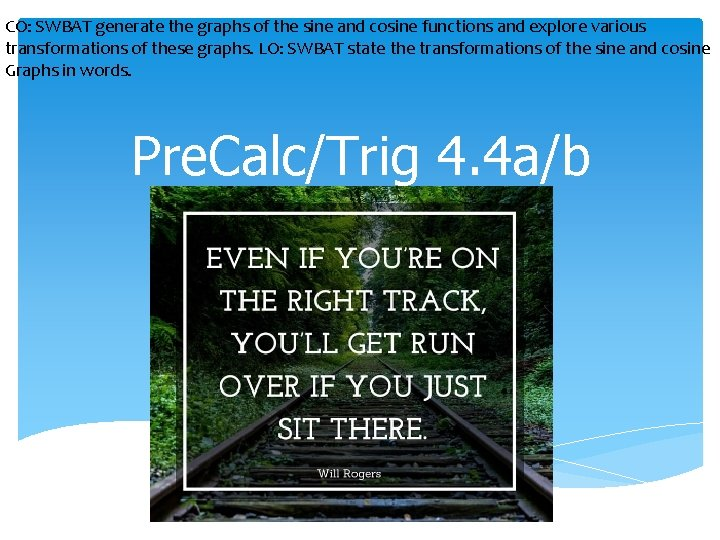CO: SWBAT generate the graphs of the sine and cosine functions and explore various transformations of these graphs. LO: SWBAT state the transformations of the sine and cosine Graphs in words. Pre. Calc/Trig 4. 4 a/b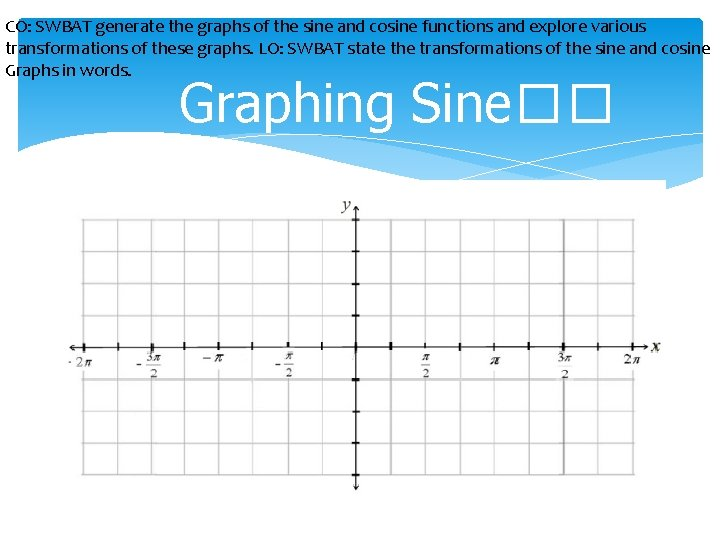CO: SWBAT generate the graphs of the sine and cosine functions and explore various transformations of these graphs. LO: SWBAT state the transformations of the sine and cosine Graphs in words. Graphing Sine��CO: SWBAT generate the graphs of the sine and cosine functions and explore various transformations of these graphs. LO: SWBAT state the transformations of the sine and cosine Graphs in words. Graphing Cosine ��CO: SWBAT generate the graphs of the sine and cosine functions and explore various transformations of these graphs. LO: SWBAT state the transformations of the sine and cosine Graphs in words. Amplitude is the height of the sinusoid from the center. It is always written as |a|.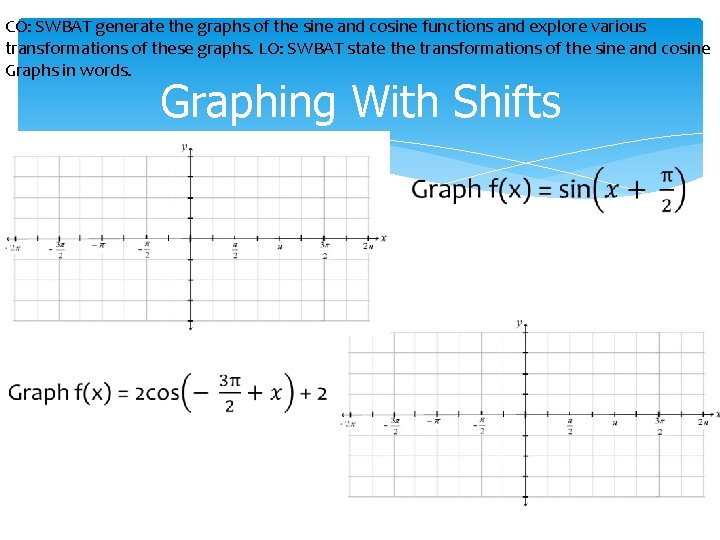CO: SWBAT generate the graphs of the sine and cosine functions and explore various transformations of these graphs. LO: SWBAT state the transformations of the sine and cosine Graphs in words. Graphing With ShiftsCO: SWBAT generate the graphs of the sine and cosine functions and explore various transformations of these graphs. LO: SWBAT state the transformations of the sine and cosine Graphs in words. Assignment: 4. 4 WS Part I and II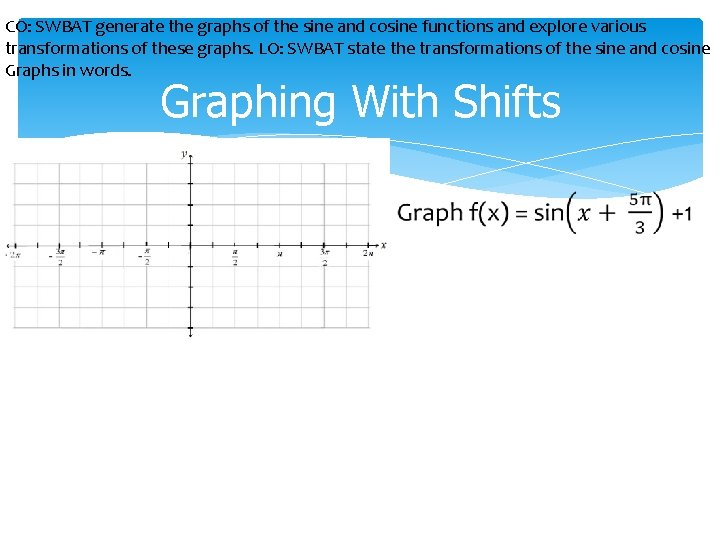CO: SWBAT generate the graphs of the sine and cosine functions and explore various transformations of these graphs. LO: SWBAT state the transformations of the sine and cosine Graphs in words. Graphing With Shifts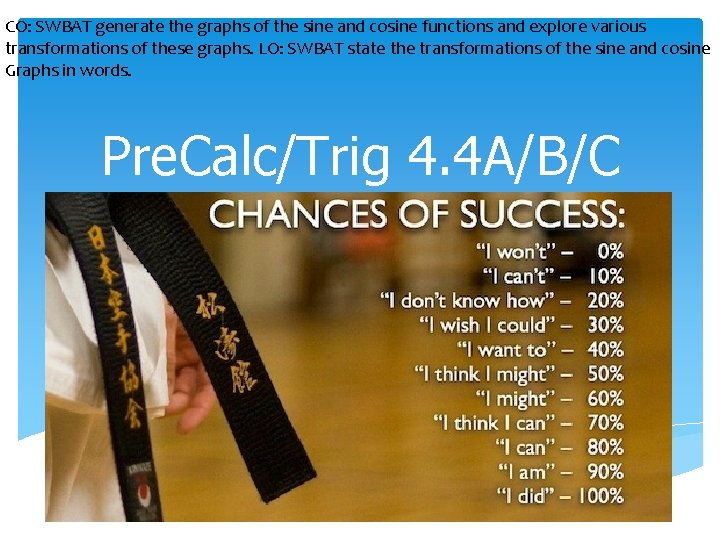CO: SWBAT generate the graphs of the sine and cosine functions and explore various transformations of these graphs. LO: SWBAT state the transformations of the sine and cosine Graphs in words. Pre. Calc/Trig 4. 4 A/B/C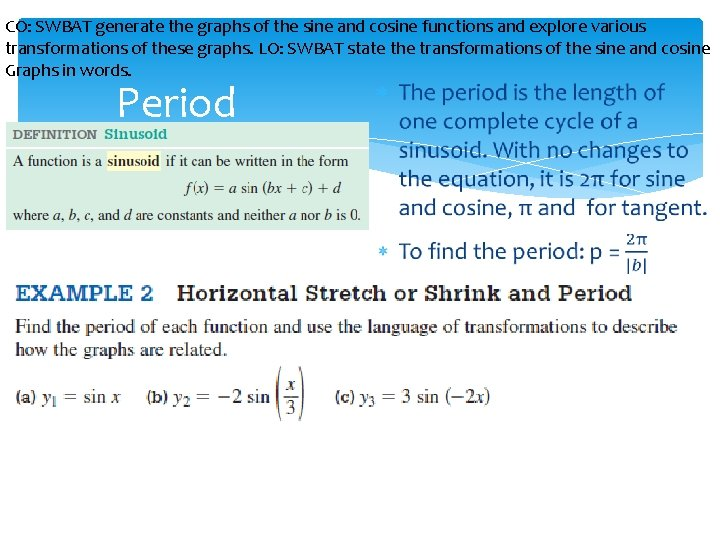CO: SWBAT generate the graphs of the sine and cosine functions and explore various transformations of these graphs. LO: SWBAT state the transformations of the sine and cosine Graphs in words. Period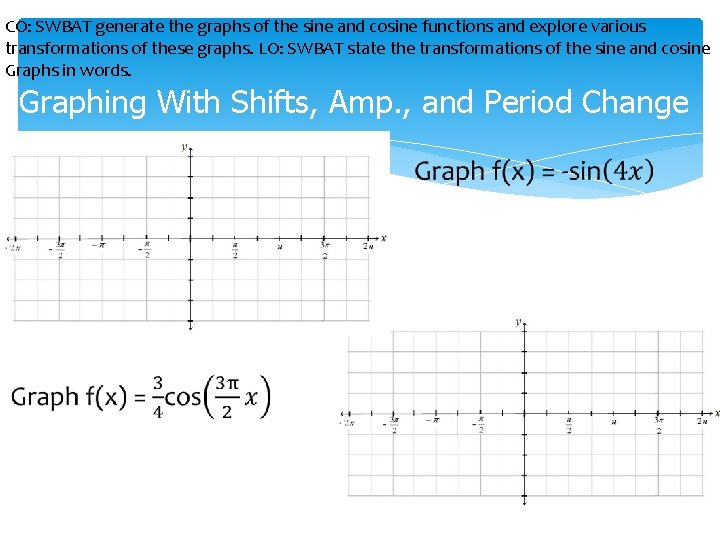CO: SWBAT generate the graphs of the sine and cosine functions and explore various transformations of these graphs. LO: SWBAT state the transformations of the sine and cosine Graphs in words. Graphing With Shifts, Amp. , and Period ChangeCO: SWBAT generate the graphs of the sine and cosine functions and explore various transformations of these graphs. LO: SWBAT state the transformations of the sine and cosine Graphs in words. Graphing With Shifts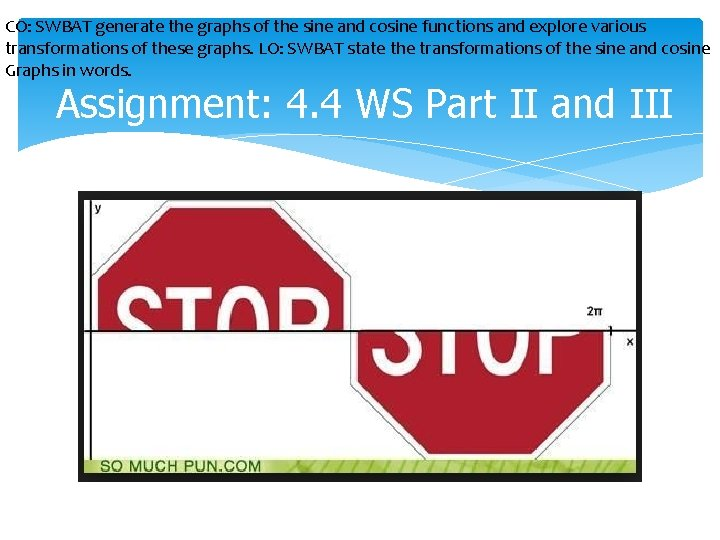CO: SWBAT generate the graphs of the sine and cosine functions and explore various transformations of these graphs. LO: SWBAT state the transformations of the sine and cosine Graphs in words. Assignment: 4. 4 WS Part II and IIICO: SWBAT generate the graphs of the sine and cosine functions and explore various transformations of these graphs. LO: SWBAT state the transformations of the sine and cosine Graphs in words. Objective Check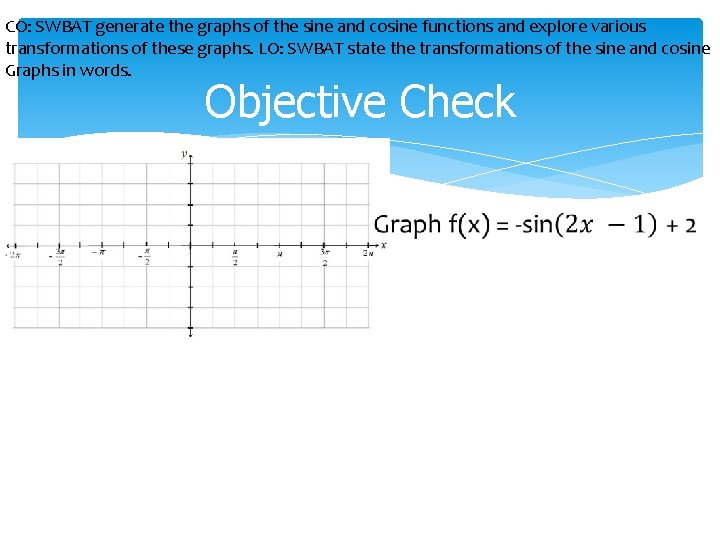CO: SWBAT generate the graphs of the sine and cosine functions and explore various transformations of these graphs. LO: SWBAT state the transformations of the sine and cosine Graphs in words. Objective CheckCO: SWBAT generate the graphs of the reciprocal functions and explore various transformations of these graphs. LO: SWBAT state the transformations of the reciprocal graphs. Pre. Calc/Trig 4. 5 a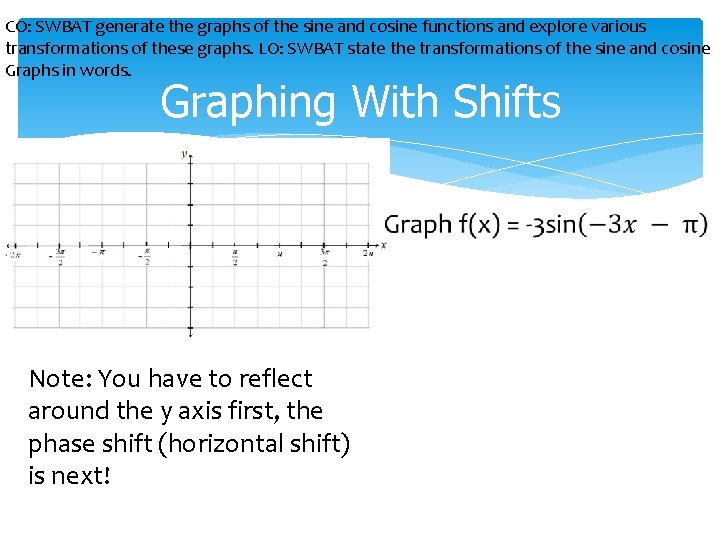CO: SWBAT generate the graphs of the sine and cosine functions and explore various transformations of these graphs. LO: SWBAT state the transformations of the sine and cosine Graphs in words. Graphing With Shifts Note: You have to reflect around the y axis first, the phase shift (horizontal shift) is next!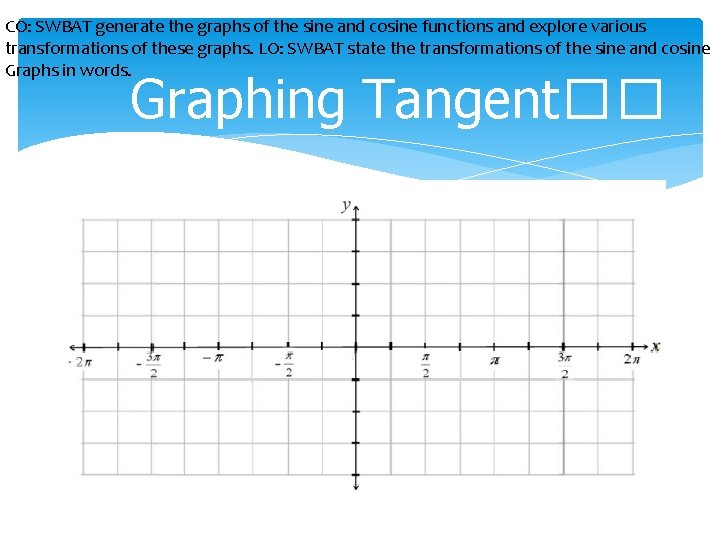CO: SWBAT generate the graphs of the sine and cosine functions and explore various transformations of these graphs. LO: SWBAT state the transformations of the sine and cosine Graphs in words. Graphing Tangent��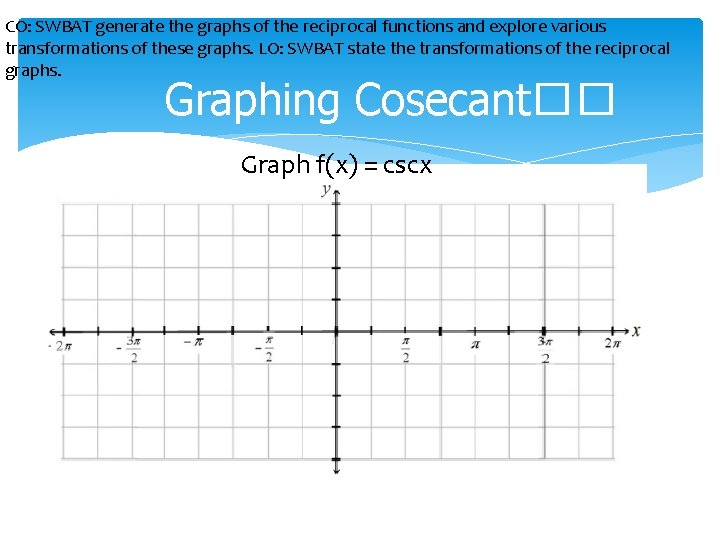CO: SWBAT generate the graphs of the reciprocal functions and explore various transformations of these graphs. LO: SWBAT state the transformations of the reciprocal graphs. Graphing Cosecant�� Graph f(x) = cscx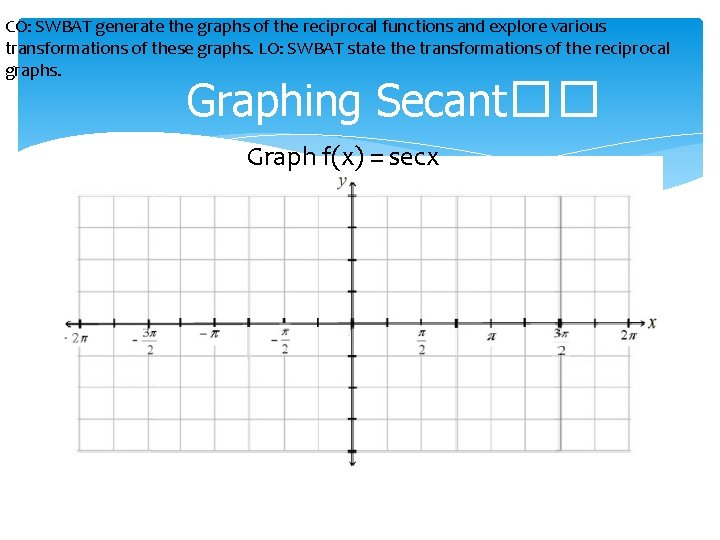CO: SWBAT generate the graphs of the reciprocal functions and explore various transformations of these graphs. LO: SWBAT state the transformations of the reciprocal graphs. Graphing Secant�� Graph f(x) = secxCO: SWBAT generate the graphs of the reciprocal functions and explore various transformations of these graphs. LO: SWBAT state the transformations of the reciprocal graphs. Graphing Cotangent�� Graph f(x) = cotxCO: SWBAT generate the graphs of the reciprocal functions and explore various transformations of these graphs. LO: SWBAT state the transformations of the reciprocal graphs. Graphing With Shifts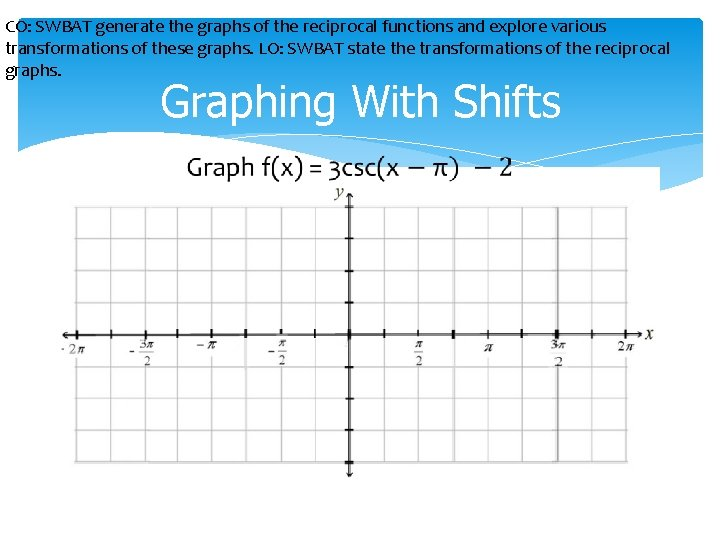CO: SWBAT generate the graphs of the reciprocal functions and explore various transformations of these graphs. LO: SWBAT state the transformations of the reciprocal graphs. Graphing With Shifts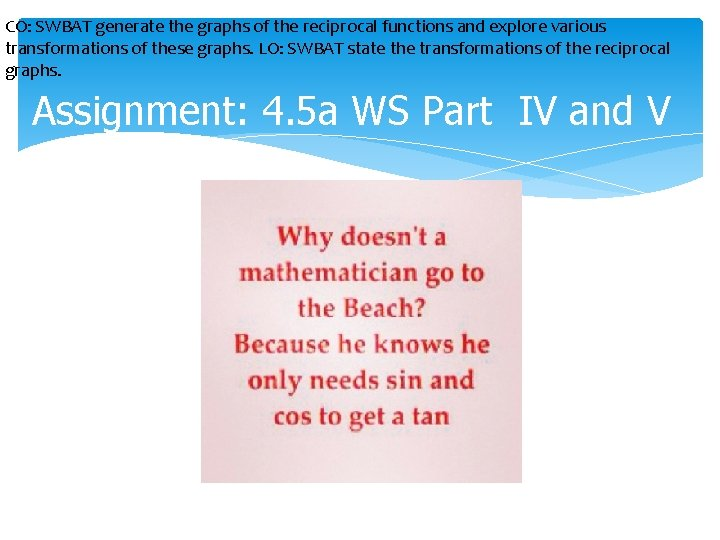CO: SWBAT generate the graphs of the reciprocal functions and explore various transformations of these graphs. LO: SWBAT state the transformations of the reciprocal graphs. Assignment: 4. 5 a WS Part IV and VCO: SWBAT generate the graphs of the reciprocal functions and explore various transformations of these graphs. LO: SWBAT state the transformations of the reciprocal graphs. Objective Check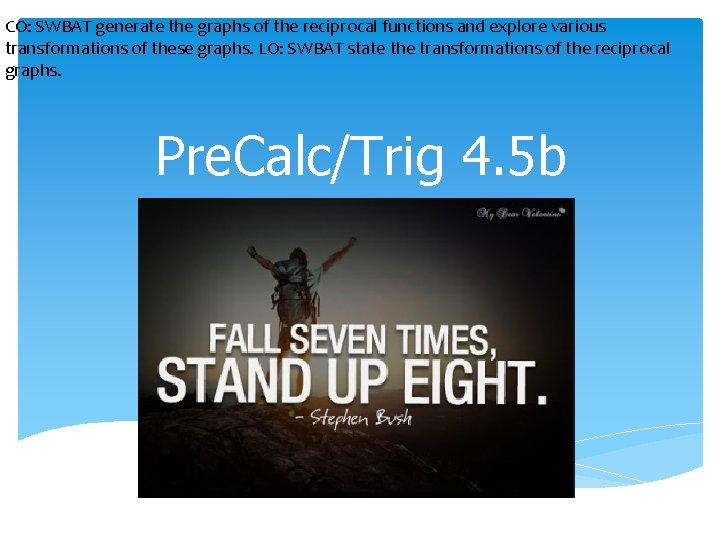CO: SWBAT generate the graphs of the reciprocal functions and explore various transformations of these graphs. LO: SWBAT state the transformations of the reciprocal graphs. Pre. Calc/Trig 4. 5 bCO: SWBAT generate the graphs of the reciprocal functions and explore various transformations of these graphs. LO: SWBAT state the transformations of the reciprocal graphs. Graphing With ShiftsCO: SWBAT generate the graphs of the reciprocal functions and explore various transformations of these graphs. LO: SWBAT state the transformations of the reciprocal graphs. Graphing With Shifts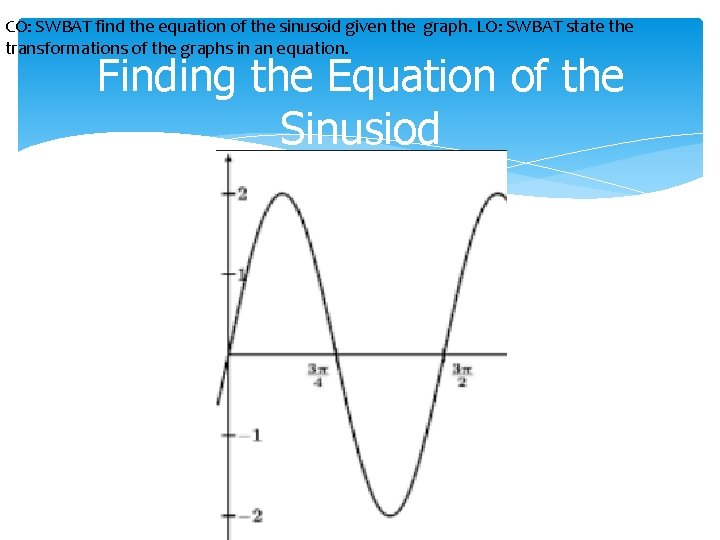CO: SWBAT find the equation of the sinusoid given the graph. LO: SWBAT state the transformations of the graphs in an equation. Finding the Equation of the Sinusiod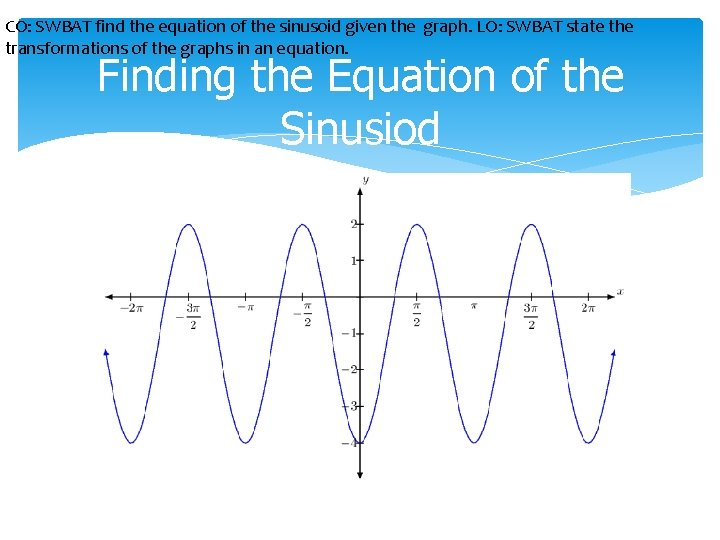CO: SWBAT find the equation of the sinusoid given the graph. LO: SWBAT state the transformations of the graphs in an equation. Finding the Equation of the Sinusiod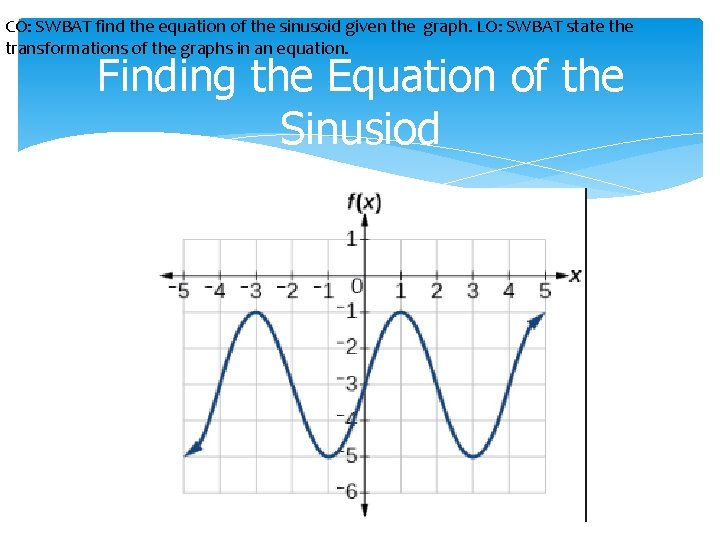CO: SWBAT find the equation of the sinusoid given the graph. LO: SWBAT state the transformations of the graphs in an equation. Finding the Equation of the SinusiodCO: SWBAT generate the graphs of the reciprocal functions and explore various transformations of these graphs. LO: SWBAT state the transformations of the reciprocal graphs. Assignment: WS 4 -3